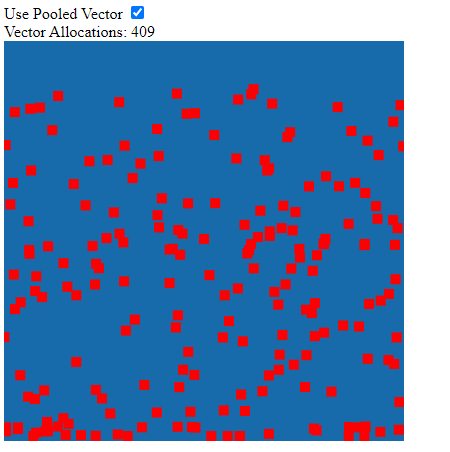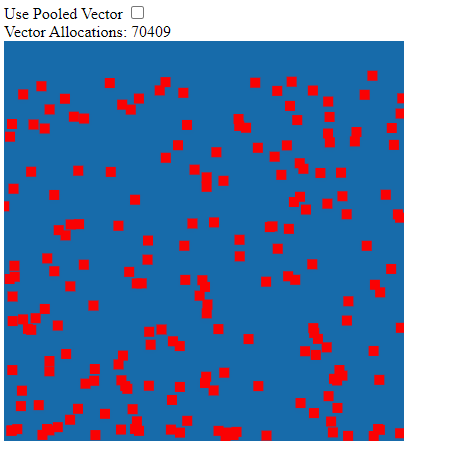# JavaScript Object Pools for Value Objects

### Published on Sunday, October 18th, 2020

What do I mean by "value" objects? I mean things that behave like numbers conceptually. For example `const result = 1 + 2`, adding two numbers doesn't change the value of `1` or `2` they are immutable, but a new value falls out the left hand side into the `result`. This is a useful property, and sometimes you want JavaScript objects to behave this way. I'll call such objects them "value" objects in this post.

## Value Objects

In the example below `one` and `two` behave like values and are immutable, `result` is a new value object, not a reference to `one` or `two` that was updated.

``class Vector {    constuctor(        public x: number,        public y: number    ) {}    add(v: Vector): Vector {        return new Vector(this.x + v.x, this.y + v.y);    }    /// More implementation hidden}const one = new Vector(1, 1);const two = new Vector(2, 2);const result = one.add(two); // one still has the value (1, 1) after the 'add' operation// two still has the value (2, 2) after the 'add' operation// result is a new "value" object that has the value (3, 3)``

Immutability helps protect against bugs, especially when working with objects that represent mathematical quantities. If the value of `one` was updated to `(3, 3)` after the `.add()`, it might be unexpected (at least to me very confusing to have a "value" object change like this)!

## The JavaScript Value Object Problem

So one downside to this type of value object is how quickly these ephemeral value objects can be created and discarded! This can build up in memory quickly and cause garbage collection pauses leading to some jankinesstm!

This might be unacceptable depending on your app. If you need high throughput and performance, like a game, value object related garbage collection could be a big problem.

One solution is to use an object pool strategy! While this technique is nothing new, it can be useful for these ephemeral value objects that might be used for intermediate calculations, this can cut down on `new Vector()` allocations by an order of magnitude!

TLDR: Object Pool Source

## ⚠ Caveats of Object Pools! ⚠

I need to offer an obligatory warning, object pooling is a memory optimization technique. It effectivley works by taking on the role of memory manager instead of the very powerful and well engineered browser. Also don't trust me because I'm writing about it, verify for yourself something will work for your usecase.

### 1. They are easy to get wrong and cause a memory leak

It's important to have a limit or perhaps a soft limit with logging in an object pool. Using a pool or implementing a pool incorrectly will just cause a memory leak that the browser cannot garbage collect anymore.

Mitigation: Add some stress tests with large numbers of objects over time to make sure pools are working as expected.

### 2. Hard to track down bugs

Using a reference to a value object that has been returned to the pool can cause bugs. Often the source of the bug is separated from where it manifested. For example if a leaked reference to a value object managed by the pool was reused and values were change it may cause invalid results in calculations.

Mitigation: Track the state of your objects and throw when used in invalid contexts.

## Value Object Pool Implementation Details

We can compare the difference in allocations with a quick modification to our `Vector` type to track the number of constructions. This type of thing is super useful in general if you're looking to increase memory efficency

``class Vector {    public static ALLOCATIONS = 0;    constuctor(        public x: number,        public y: number    ) {        Vector.ALLOCATIONS++;    }    //...}``

In order for this type of value object to make full use of the pool we need to pollute the type with some pool logic 😱 A less effecient version could be done without doing this. I try to minimize the intrusiveness of this change with a `_new(x: number, y: number)` helper to abstract the pooling decision. Send me a note if you have any other suggestions on how to accomplish this better!

``interface Poolable<Type = any> {    _pool?: Pool<Type>;}// Implements a `Poolable` interface which decorates a `_pool` propclass Vector implements Poolable<Vector> {    static _ALLOCATIONS = 0;    //     public _pool?: Pool<Vector> = undefined;    private _new(x: number, y: number): Vector {        return this._pool?.get(x, x) ?? new Vector(x, x);    }    //...    add(v: Vector): Vector {        return this._new(this.x + v.x, this.y + v.y);    }    //...}``

With our `Vector` value object setup to make use of pooling, we can use `using(...)` to do all of our intermediate calculations, then when the using block is done all of the vectors used are recycled! This means we only need enough unique vectors to perform the calculation, none of the instances get waisted.

``const VectorPool = new Pool<Vector>(    // Builder Function tells the pool how to build an object    (x, y) => new Vector(x, y),    // Recycler Function tells the pool how to update an object for re-use    (v, x, y) => { v.x = x; v.y = y; return v; },    // Max size on a pool is important to help catch memory leaks    100)// Update 200 things in my game loopfor (let i = 0; i < entities.length; i++) {    // After the using all Vectors are reclaimed for later use!    const [pos, vel] = VectorPool.using((p: Pool<Vector>) => {        // Uses the builder or recycler to retrieve a vector instance        const position = p.get().copyFrom(entities[i].pos);        const velocity = p.get().copyFrom(entities[i].vel);        const acceleration = p.get(0, accel);        // Perform euler position approximation        const finalVelocity = velocity.add(acceleration.scale(time));        const finalPosition = position.add(velocity.scale(time)).add(acceleration.scale(.5 * time * time));        entities[i].pos.copyFrom(finalPosition);        entities[i].vel.copyFrom(finalVelocity);        // Returning these vectors from the pool `using()` signals that these will be unmanaged        // These vectors are taken out of the pool        return [finalPosition, finalVelocity]    });}``

## Object Pool Example & Results

The results are really impressive for the slightly contrived example of a game type program below! It becomes pretty clear the amount of objects that can be generated over time in this type of scenario if not pooled.

Here is the source for the object pool if you want to use it for yourself!

### Pooled### Non-pooledMore allocations can be squeezed if no pooled value objects are allowed to leave the pool with a `using()` or `done()`. Organizing your internal calculation this way can produce some very memory efficent results 😎

Hope this helps the person out there trying to do the same thing!

Cheers,

-Erik

Help support me on Github Sponsors or Patreon

Become a Patron!